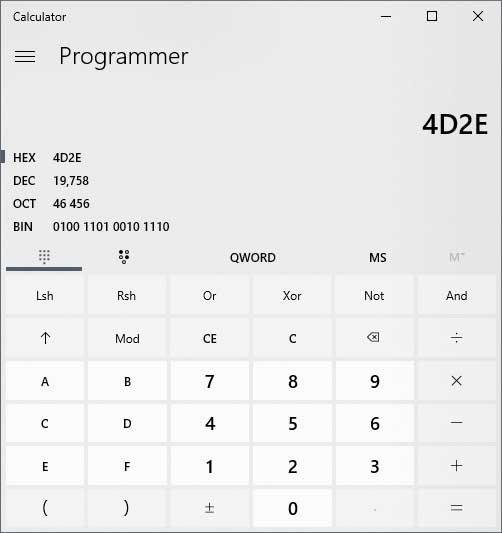### What the Hex is Going on Here?

You may or may not have heard the term ones and zeros when people talk about computers or try to act "techy". What they are referring to is the binary numbering system which computers use to function with 1 representing on and 0 representing off. An example of a binary number might look like 0110010110.

But binary numbers aren’t the only game in town. There is another numbering system that is use by computers that serves a similar purpose and that is the hexadecimal numbering system or hex for short. With the increased usage of IPv6 addresses you will be seeing even more of the hexadecimal numbering system if you are an IT administrator so now is the time to get used to it.

In the computing world, hexadecimal numbering is used for such things as MAC addresses and memory addresses. It is used to represent large numbers in a smaller, easier to read format. An example of a hexadecimal number would look something like 2FE4C0 which is 001011111110010011000000 in binary value. As you can see it’s a lot easier to read, remember or write down 2FE4C0 compared to 001011111110010011000000! The hex number 2FE4C0 can also be represented as 3,138,752 in decimal value. Here is a table that shows the conversions for the 16 hex values to binary and to decimal.

 Hexadecimal Binary Decimal 0 0000 0 1 0001 1 2 0010 2 3 0011 3 4 0100 4 5 0101 5 6 0110 6 7 0111 7 8 1000 8 9 1001 9 A 1010 10 B 1011 11 C 1100 12 D 1101 13 E 1110 14 F 1111 15

The hexadecimal numbering system is a base 16 system divided up into groups of 4 bits (called a nibble), and since we are used to 0-9 for our decimal numbering system we use the letters A-F to represent the numbers from 11 to 15 to make up 16 values in total (0-F). The word hexadecimal actually translates to the word sixteen. As you can see in the table above that the hexadecimal values go from 0 to F and the decimal equivalents go from 0 to 15. You might have noticed that a 4 digit binary number can be represented by one hex number which can greatly reduce how long a value representation needs to be.

The reason hexadecimal numbers are able to be used to represent very large numbers is because each value in the hex number is worth 16 times as much as the value to the right of it compared to 10 times the value with the base 10 decimal system. And yes the values increment from right to left just like with binary (which is a base 2 system) so it will look something like 167 166 165 164 163 162 161 160 and so on. As you can see these values can get pretty large the more left you go.

160 = 1
161 = 16
162 = 256
163 = 4096
164 = 65536
165 = 1048576
166 = 16777216
167 = 268435456

So what happens if you want to go higher than the decimal value of 15? In that case the chart would start to look like this.

 Decimal Hexadecimal Binary 15 F 1111 16 10 0001 0000 17 11 0001 0001 18 12 0001 0010 19 13 0001 0011 20 14 0001 0100 21 15 0001 0101 22 16 0001 0110 23 17 0001 0001 24 18 0001 0010 25 19 0001 0011 26 1A 0001 1010 27 1B 0001 1011 28 1C 0001 1100 29 1D 0001 1101 30 1E 0001 1110 31 1F 0001 1111 32 20 0010 0000

Keep in mind that just because decimal 16 is displayed as 10 doesn’t mean that it’s actually the number 10 but actually 1+0 and at the same time the decimal number 17 is really 1+1. This makes a little more sense if you compare the hex number to its binary equivalent. For example 1+0 in binary is really 0001 (for 1) and 0000 (for 0). As for the number 26 which is represented as 1A in hex, think of it as 1+10 since the decimal value for 1 is 1 in hex and the decimal value for 10 is A in hex and the hex numbering system goes 0-9 then starts at A-F so A comes after the 9 in 19. Then 1 in binary is 0001 and A in binary is 1010.

Hexadecimal numbering can be confusing just like binary numbering but once you play around with it a little and compare hex to binary to decimal things will make a little more sense. After you have the basic understanding you can then use online calculators to do your conversions or even the Windows calculator in programmer mode.As you can see you can change the numbering scheme to the type you want such as hec, dec, oct or bin and then enter your number and it will convert it to all the other values making it easy to do the translations.